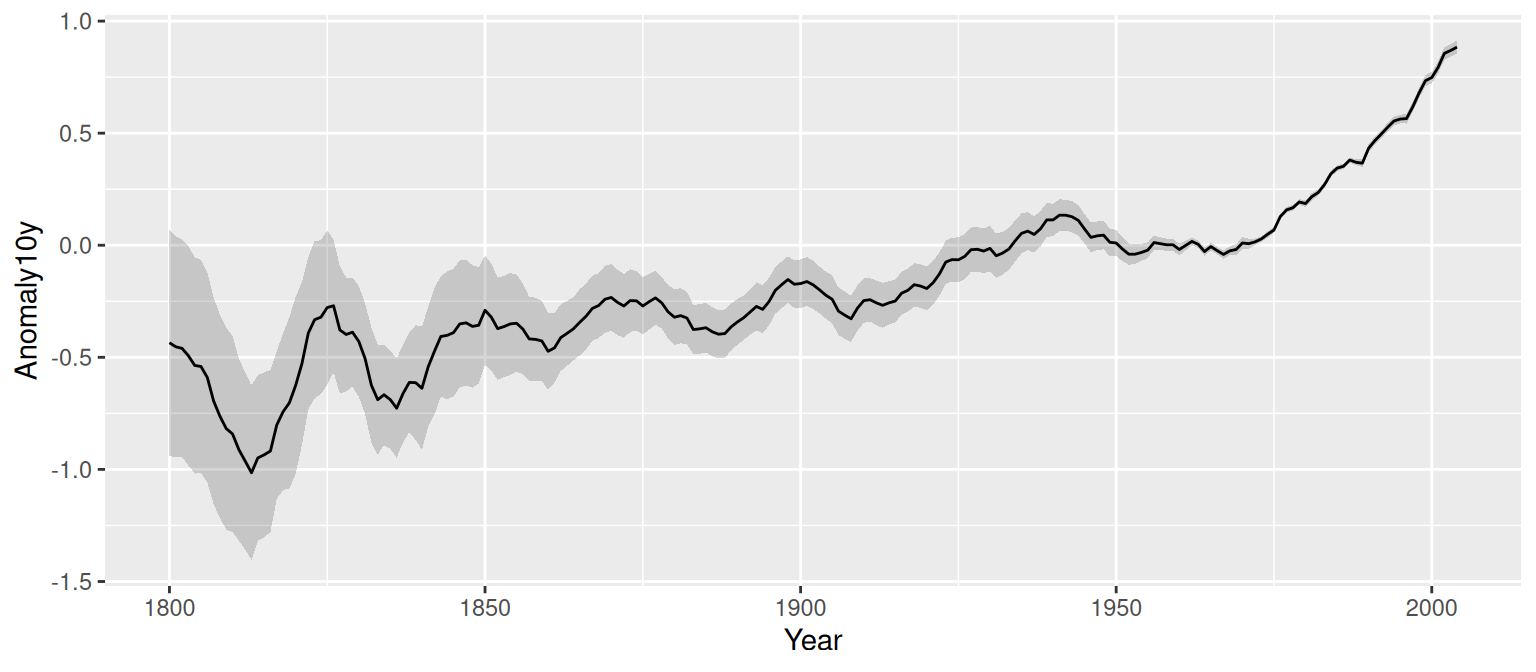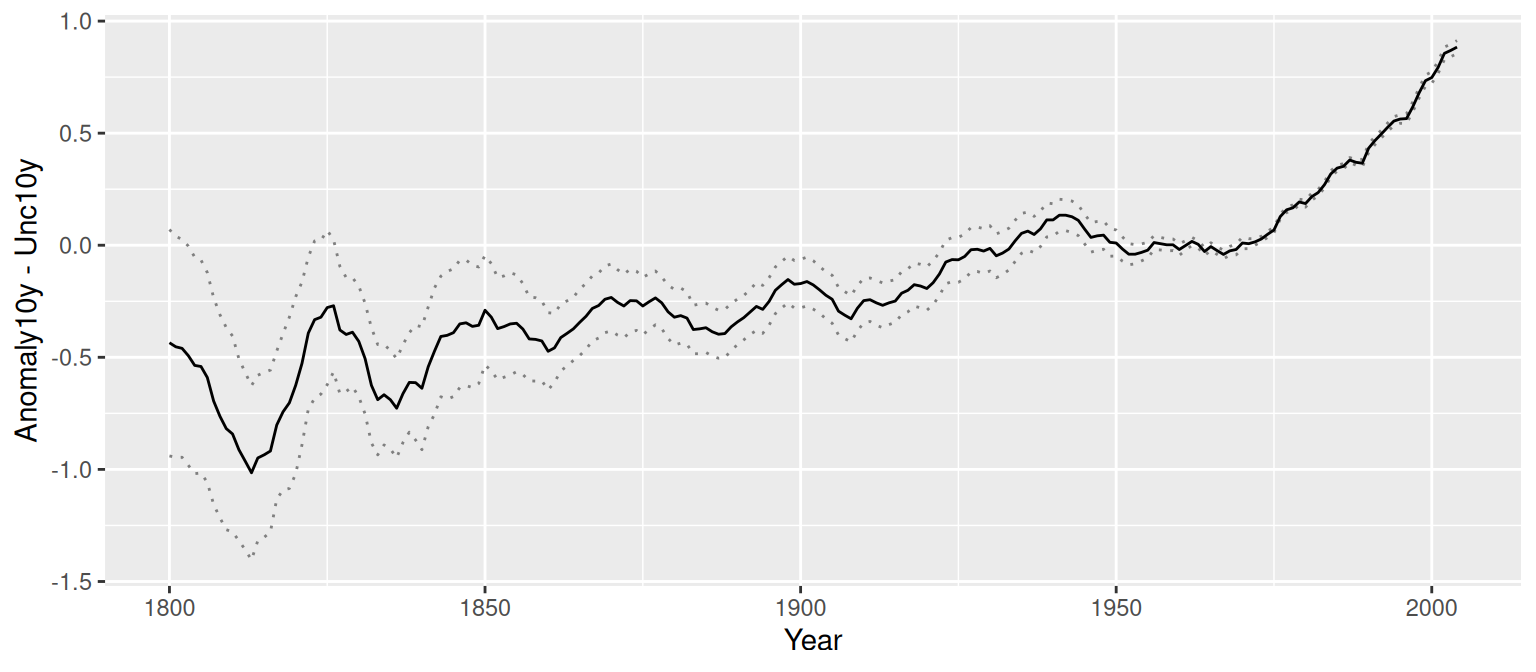## 4.9 Adding a Confidence Region

### 4.9.1 Problem

You want to add a confidence region to a graph.

### 4.9.2 Solution

Use `geom_ribbon()` and map values to `ymin` and `ymax`.

In the `climate` data set, `Anomaly10y` is a 10-year running average of the deviation (in Celsius) from the average 1950–1980 temperature, and `Unc10y` is the 95% confidence interval. We’ll set `ymax` and `ymin` to `Anomaly10y` plus or minus `Unc10y` (Figure 4.24):

``````library(gcookbook) # Load gcookbook for the climate data set
library(dplyr)

# Grab a subset of the climate data
climate_mod <- climate %>%
filter(Source == "Berkeley") %>%
select(Year, Anomaly10y, Unc10y)

climate_mod
#>     Year Anomaly10y Unc10y
#> 1   1800     -0.435  0.505
#> 2   1801     -0.453  0.493
#> 3   1802     -0.460  0.486
#>  ...<199 more rows>...
#> 203 2002      0.856  0.028
#> 204 2003      0.869  0.028
#> 205 2004      0.884  0.029

ggplot(climate_mod, aes(x = Year, y = Anomaly10y)) +
geom_ribbon(aes(ymin = Anomaly10y - Unc10y, ymax = Anomaly10y + Unc10y), alpha = 0.2) +
geom_line()``````Figure 4.24: A line graph with a shaded confidence region

The shaded region is actually a very dark grey, but it is mostly transparent. The transparency is set with `alpha = 0.2`, which makes it 80% transparent.

### 4.9.3 Discussion

Notice that the `geom_ribbon()` comes before `geom_line()`, so that the line is drawn on top of the shaded region. If the reverse order were used, the shaded region could obscure the line. In this particular case that wouldn’t be a problem since the shaded region is mostly transparent, but it would be a problem if the shaded region were opaque.

Instead of a shaded region, you can also use dotted lines to represent the upper and lower bounds (Figure 4.25):

``````# With a dotted line for upper and lower bounds
ggplot(climate_mod, aes(x = Year, y = Anomaly10y)) +
geom_line(aes(y = Anomaly10y - Unc10y), colour = "grey50", linetype = "dotted") +
geom_line(aes(y = Anomaly10y + Unc10y), colour = "grey50", linetype = "dotted") +
geom_line()``````Figure 4.25: A line graph with dotted lines representing a confidence region

Shaded regions can represent things other than confidence regions, such as the difference between two values, for example.

In the area graphs in Recipe 4.7, the y range of the shaded area goes from 0 to y. Here, it goes from `ymin` to `ymax`.